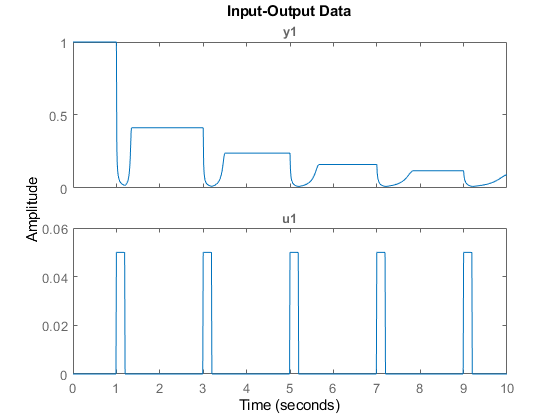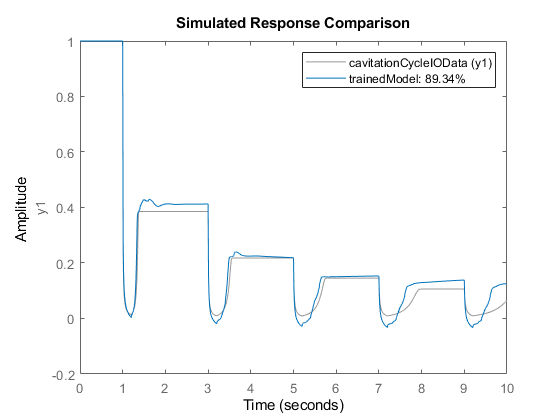# Surrogate Modeling Using Gaussian Process-Based NLARX Model

In this example, you replace a hydraulic cavitation cycle model in Simulink® with a surrogate nonlinear ARX (NLARX) model to facilitate faster simulation.

In the hydraulic cavitation cycle for this example, the fluid in a custom double-acting cylinder can cavitate and recover. During the repeating cycle of oscillating pressure, the absolute pressure does not drop below absolute zero as the bulk modulus of the homogeneous liquid-gas mixture decreases accordingly at low pressures. The input to this system is a velocity source, and the output observed is the absolute pressure in the cylinder chambers.

Often, such physical models are computationally expensive to simulate. You can reduce simulation time by replacing the model with a simpler surrogate model. In this example, you create a surrogate model for this physical system an estimated NLARX model with a Gaussian process nonlinear output function. Using this approach, you can guide the dynamics of the system by choosing how regressors (delayed forms of input-output data) interact with the nonlinear output function.

### Obtain Input-Output Data

To estimate the model, you must first obtain input-output data by simulating the original model.

Open the model and set the amplitude of the velocity input signal.

```modelName = 'HydraulicCavitationCycleFullModel'; velocityAmplitude = 0.05; %#ok<*NASGU> open_system(modelName)```Model estimation requires an input signal that is rich enough to capture the dynamics of this nonlinear system. Therefore, the model applies a pulse train velocity signal to the custom cylinder.

This model includes an Iddata Sink block, which saves the simulated input-output data to the MATLAB® workspace as an `iddata` object.

Simulate the model.

`sim(modelName);`

Plot the data from the saved `iddata` object.

`plot(cavitationCycleIOData)`Here, y1 is the output signal, that is the absolute pressure in the cylinder chambers. `u1` is the input velocity applied to the custom cylinder.

This system is sensitive to changes in the input source. You must take this sensitivity into account when you train, validate, and use the NLARX model.

### Estimate Model

Next, you estimate an NLARX model using this input-output data. The goal is to reasonably replicate the dynamics of the cavitation cycle.

An important aspect of NLARX estimation is the choice of nonlinear output function. For this example, you use a Gaussian process output function. The versatility in kernel function provided by this output function allows for variability in model structure.

Create a Gaussian process mapping object with default settings.

`gpObject = idGaussianProcess;`

Create a regressor set for the NLARX model.

```vars = {'y1','u1'}; lags = {1:30,1:60}; regressors = linearRegressor(vars,lags);```

Estimate a NLARX model using the Gaussian process output function and the defined linear regressors.

`trainedModel = nlarx(cavitationCycleIOData,regressors,gpObject)`
```trainedModel = Nonlinear ARX model with 1 output and 1 input Inputs: u1 Outputs: y1 Regressors: Linear regressors in variables y1, u1 List of all regressors Output function: Gaussian process function using a SquaredExponential kernel. Sample time: 0.01 seconds Status: Estimated using NLARX on time domain data "cavitationCycleIOData". Fit to estimation data: 99.96% (prediction focus) FPE: 1.586e-08, MSE: 1.223e-08 ```

### Validate Model

To check the performance of the trained model, first obtain validation data from the original Simulink model. For this example, increase the velocity amplitude by 4% and simulate the model.

```velocityAmplitude = 1.04*0.05; sim(modelName);```

Compare the output of the trained model to the simulation output using the validation data.

`compare(cavitationCycleIOData,trainedModel)`The model fit for the validation data is over 95%, which indicates that the trained model reasonably represents the dynamics of the system.

### Test Model Sensitivity

The performance of the trained model is sensitive to the amplitude of the velocity input. To demonstrate this sensitivity, simulate the trained system with a larger velocity amplitude.

```velocityAmplitude = 1.1*0.05; sim(modelName);```

Compare the performance of the trained model to the simulation using this testing data.

`compare(cavitationCycleIOData,trainedModel)`For this data, the fit for the trained model is below 90% and the output pressure differs significantly, particularly for lower pressure values.

Given the sensitivity of the trained model, you can consider training another surrogate model for larger amplitude operating conditions while retaining the existing regressor configuration with sufficiently good fit. Doing so demonstrates the flexibility of this modeling approach.

### Substitute Surrogate Model

To substitute the surrogate system into the Simulink model, use a Nonlinear ARX Model block. As an example, open the `GPsurrogateModel` Simulink model.

```surrogateModelName = 'GPSurrogateModel'; open_system(surrogateModelName)```Simulate the surrogate model using the same velocity amplitude as the original estimation data.

```velocityAmplitude = 0.05; gpSurrogateModelData = sim(surrogateModelName);```

Simulate the original model using the same velocity amplitude.

`sim(modelName);`

Plot the simulation output and compare it with the estimation data output.

```plot(gpSurrogateModelData.tout,gpSurrogateModelData.simout, ... cavitationCycleIOData.SamplingInstants,cavitationCycleIOData.OutputData); title('Surrogate Model Performance - Estimation Data') xlabel('Time (s)') ylabel('Absolute Pressure (atm)') legend('Surrogate model','Original model')```## SupportGet trial now